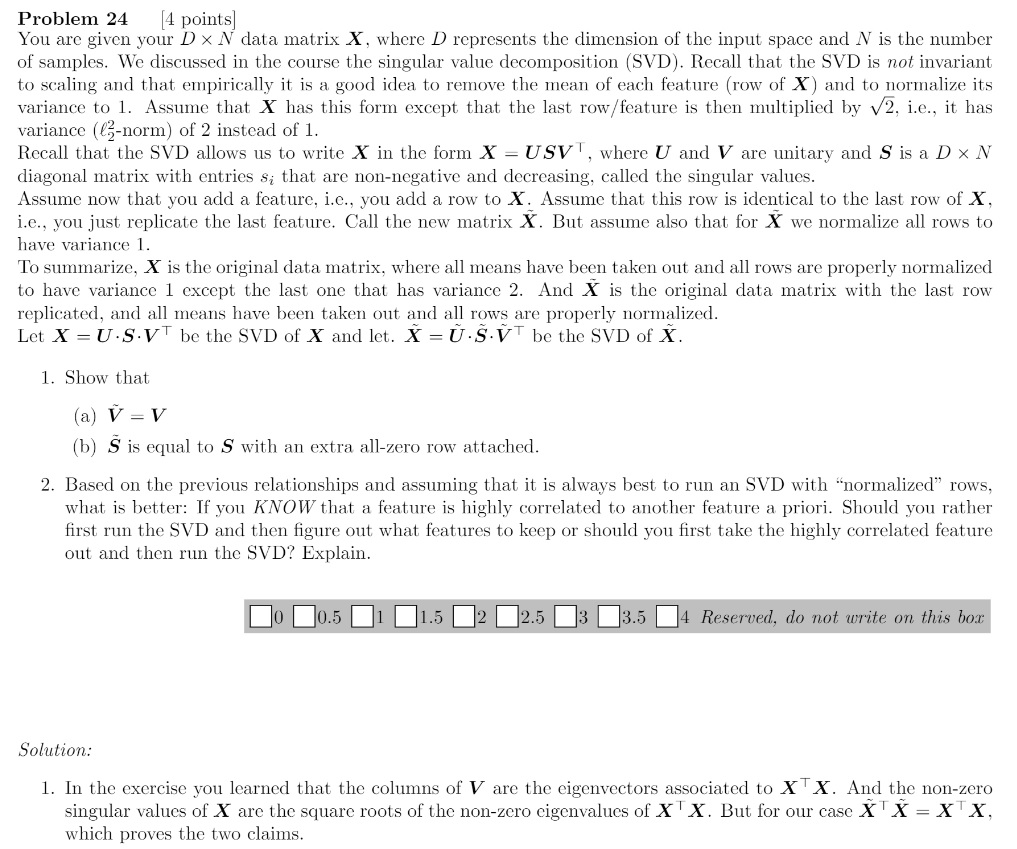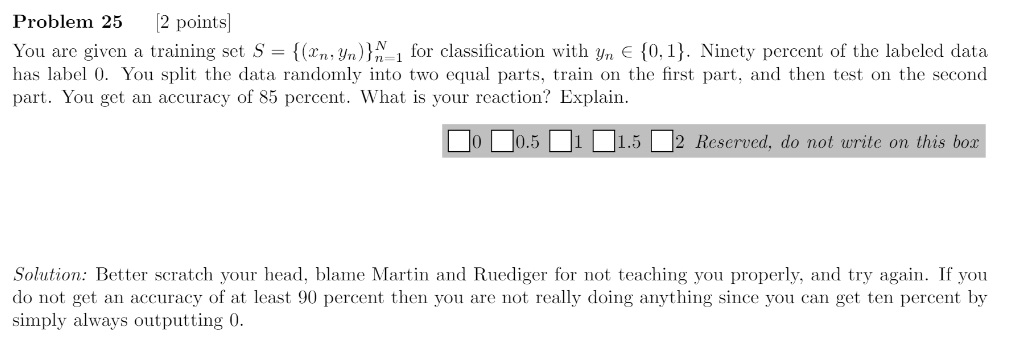### 2017 Q24 possible error + Q25

Hi,X.T @ X has dimension NN whereas the other has (N+1)(N+1). I think the solution should be that X @ X.T and X~ @ X~.T are the same, thus they have the same eigendecomposition. In the case of X~ @ X~.T, S~ has an additionnal row. In order to keep the same value, this row should be equal to 0. Then we can deduce V from U.For this answer, instead of blaming the prof doing his best to minimize the loss function L(grades) = 1/N sum_n = 1 to N (Indicator(grade_n < 4)), could it be caused by a bad setting of the parameters or the presence of outliers ? Perhaps we should also preserve the distribution in the training set and the testing set and normalize the data. Maybe we also need to use a feature expansion.

You have added a new feature means you have increased D to D+1, N stays unchanged. So there is nothing wrong with the solution.

For the second part, the main idea is unbalanced datasets and that accuracy is not a good measure in this case as you can get very high accuracy by always outputting the dominant class. To treat this problem you need to balance your dataset, in practice subsampling is used either oversampling the "smaller" class or oversampling the bigger one.

Page 1 of 1